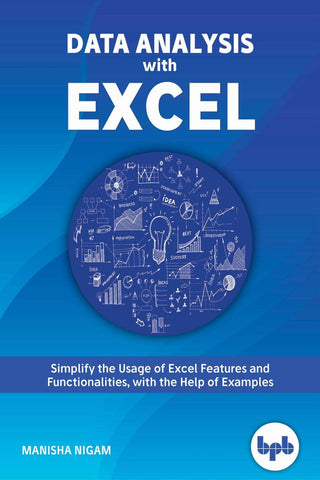# Top 8 Excel Data Analysis Functions

Data analysis is a process of cleaning, transforming, and modeling data to discover meaningful information for better decision making. Microsoft Excel is one of the top tools for Data Analysis. Excel has some powerful functions and formulas that improve your ability to analyze data.

So without wasting more time, let’s look at some Excel functions which are widely used for Data Analysis.

Sort & Filter

There may be cases where you want to work with a certain group of data while working on a large dataset. Sort function will come handy then.

Shortcut keys - ALT + H + S

Filtering

You can easily narrow down your data into subgroups with ease using this function.

Shortcut keys - ALT + H + S + F

Conditional Formatting

This function is important and used to separate important data from the remaining data. You can get the desired data based on the entered logic or condition.

Shortcut keys - ALT + H + L

Pivot Tables

Pivot Tables are the most important function used by data analysts. It helps you to sort, group, summarize and analyze data with ease.

Shortcut keys - ALT + N + V + C

CONCATENATE

CONCATENATE is another important function as it helps you combine text, numbers, and dates from different cells into one.

Formula = CONCATENATE (cells you want to combine)

VLOOKUP

VLOOKUP is one of the most useful data analysis functions. It is used to find things in a table or a range by row.

Formula =VLOOKUP(lookup_value, table_array, col_index_num, [range_lookup])

What’s Next!

If you are looking to learn different ways and methods to consolidate data, complex analysis, and prediction or forecast based on trends, then check out this book “Data Analysis with Excel.”What will you learn?

• Performing functions with shortcut keys.
• Formulas and functions
• Data visualization with new chart types
• Gantt and Milestone Chart
• Smart Art and Organization Chart
• Putting data in perspective with Pivots
• Complex data analysis using ToolPak
• Forecasting in Excel
• Mail Merge using Excel
• Macros in Excel
• What is in Excel 2019

By the end, you will get familiar with Excel Formulas for Data Analysis. You will also understand what Excel skills a data analyst needs to know. To sum things up, you will be able to leverage the power of Excel for data analysis.

## Leave a comment

All comments are moderated before being published